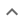•# Simplification or other simple results

We think you wrote:

###This solution deals with simplification or other simple results.

Solution found9a^4b^3

## Step by Step Solution## Step  1  :

            b2
Simplify   ——
2


#### Equation at the end of step  1  :

                  b2
(((18 • (a3)) • ——) • a) • b
2
Step  2  :

#### Equation at the end of step  2  :

               b2
(((2•32a3) • ——) • a) • b
2


## Step  3  :

#### Canceling Out :

Canceling out  2  as it appears on both sides of the fraction line

#### Equation at the end of step  3  :

  (9a3b2 • a) • b
Step  4  :

#### Multiplying exponential expressions :

b2 multiplied by b1 = b(2 + 1) = b3

## Final result :

  9a4b3